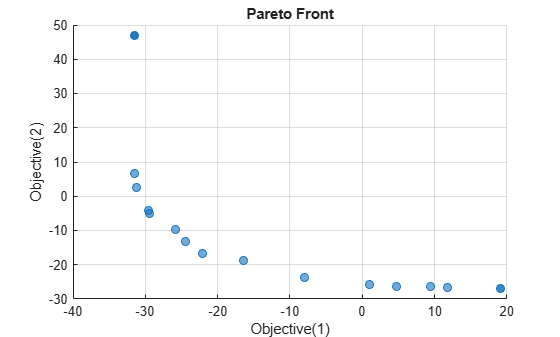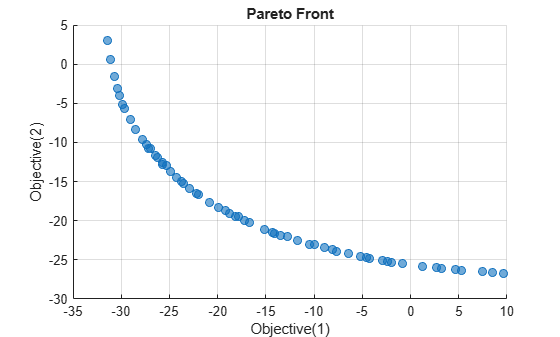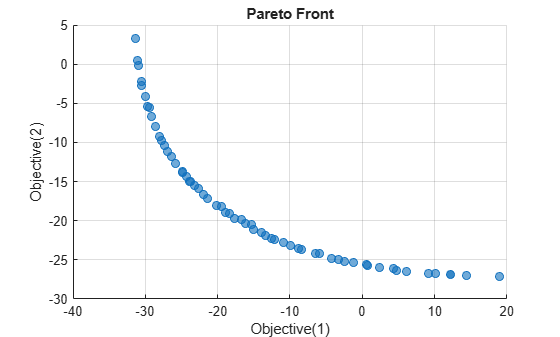# Pareto Front for Multiobjective Optimization, Problem-Based

This example shows how to solve a multiobjective optimization problem using optimization variables, and how to plot the solution.

### Problem Formulation

The problem has a two-dimensional optimization variable and two objective functions. Create the optimization variable `x` as a row vector, the orientation expected by multiobjective solvers. Set bounds specifying that the components of `x` range from –50 through 50.

`x = optimvar("x",1,2,LowerBound=-50,UpperBound=50);`

Create the two-component objective function. Include the objective function in an optimization problem.

```fun(1) = x(1)^4 + x(2)^4 + x(1)*x(2) - x(1)^2*x(2)^2 - 9*x(1)^2; fun(2) = x(1)^4 + x(2)^4 + x(1)*x(2) - x(1)^2*x(2)^2 + 3*x(2)^3; prob = optimproblem("Objective",fun);```

Review the problem.

`show(prob)`
``` OptimizationProblem : Solve for: x minimize : ((((x(1).^4 + x(2).^4) + (x(1) .* x(2))) - (x(1).^2 .* x(2).^2)) - (9 .* x(1).^2)) ((((x(1).^4 + x(2).^4) + (x(1) .* x(2))) - (x(1).^2 .* x(2).^2)) + (3 .* x(2).^3)) variable bounds: -50 <= x(1) <= 50 -50 <= x(2) <= 50 ```

### Solve and Plot Solution

Call `solve` to solve the problem.

```rng default % For reproducibility sol = solve(prob)```
```Solving problem using gamultiobj. Optimization terminated: average change in the spread of Pareto solutions less than options.FunctionTolerance. ```
```sol = 1x18 OptimizationValues vector with properties: Variables properties: x: [2x18 double] Objective properties: Objective: [2x18 double] ```

Plot the resulting Pareto front.

`paretoplot(sol)`Solve the problem again using the `paretosearch` solver.

`sol2 = solve(prob,Solver="paretosearch");`
```Solving problem using paretosearch. Pareto set found that satisfies the constraints. Optimization completed because the relative change in the volume of the Pareto set is less than 'options.ParetoSetChangeTolerance' and constraints are satisfied to within 'options.ConstraintTolerance'. ```
`paretoplot(sol2)`Using default options, the `paretosearch` solver obtains a denser set of solution points than `gamultiobj`. However, `gamultiobj` obtains a wider range of values.

### Start from Single-Objective Solutions

To attempt to achieve a better spread of solutions, find the single-objective solutions starting from `x = [1 1]`.

```x0.x = [1 1]; prob1 = optimproblem("Objective",fun(1)); solp1 = solve(prob1,x0);```
```Solving problem using fmincon. Local minimum possible. Constraints satisfied. fmincon stopped because the size of the current step is less than the value of the step size tolerance and constraints are satisfied to within the value of the constraint tolerance. ```
```prob2 = optimproblem("Objective",fun(2)); solp2 = solve(prob2,x0);```
```Solving problem using fmincon. Local minimum found that satisfies the constraints. Optimization completed because the objective function is non-decreasing in feasible directions, to within the value of the optimality tolerance, and constraints are satisfied to within the value of the constraint tolerance. ```

Prepare the single-objective solutions as an initial point for `solve`. Each point must be passed as a column vector to the `optimvalues` function.

`start = optimvalues(prob,"x",[solp1.x' solp2.x']);`

Solve the multiobjective problem with `paretosearch` starting from the `start` points.

`sol3 = solve(prob,start,Solver="paretosearch");`
```Solving problem using paretosearch. Pareto set found that satisfies the constraints. Optimization completed because the relative change in the volume of the Pareto set is less than 'options.ParetoSetChangeTolerance' and constraints are satisfied to within 'options.ConstraintTolerance'. ```
`paretoplot(sol3)`This time, `paretosearch` finds a larger range of the objective functions, going almost to 10 in Objective 2 and almost to 20 in Objective 1. This range is similar to the `gamultiobj` range, except for the anomalous solution point near Objective 1 = –31, Objective 2 = 48.